Algebraic General Topology. Vol 1: Paperback / E-book || Axiomatic Theory of Formulas: Paperback / E-book

As an Amazon Associate I earn from qualifying purchases.

I’m not a profe s sional mathematician, I work as a pro-
grammer.
I have been studying in a university in Russia but have not
ﬁnished my study.
So, I know little beyond my specia lization.
Neverth eless in my free time I discovered a n ew theory which
would com pletely overturn gen er al topology.
1
In this lesson I present my discovery, the theory of funcoids
and reloid s .
I will not give here proofs of my results, a s you can read my
actual ar t icles if you get interested in know in g the details.
The motivation for study of funcoids and reloi ds is th at
they are an elegant generalization of “spaces” (topological,
pretopological, proximity, uniform spaces) and of binary
relations between ele ments of spaces.
For brevity I will be sometimes a li ttle inf o rmal in this lesson,
for instance co nsidering compositi o n of fun coids (see below)
withou t explicitly formulating that they a re compo s able.
2
What is Algebraic General Topology?
I have introduced and r es earched objects called fun coids,
reloids, and their generalizations.
I have named the theor y of these objects Algebraic Genera l
Topology.
See
http://www.mathematics21.org
3
My gener alizati ons
Funcoids are a gener a lization of:
proximity spaces
pretopological spaces
preclos ures
digraphs (that is bin a ry relations)
Reloids are a generalization o f:
unifor m spaces
digraphs (that is bin a ry relations)
4
Usage of f uncoids and reloids
That funcoid s and reloids are a common ge neralization of
spaces and functions (functions are a special case of b in ary
relations), it makes the m a smart tool for expressing prop-
erties of fun ct ions in reg a rd of spaces .
For example, the stateme nt f is a continu o us function from
a space µ to a space ν can be expressed by the formula:
f µ ν f .
Algebraic General Topolog y is a generalization of customary
general topology but is mu ch more elegant than the cus-
tomary general topology.
5
Filters
The theory of funcoids and reloids is based on the th eory of
ﬁlters.
I’ve written an article on the theory of ﬁlters and their gen-
eralizations:
http://www.mathematics21.org
In that article I consid er ﬁlters on arbit rary posets and gen-
eralizations thereof. But in this lecture we will cons id er only
ﬁlters on the latt ice of subs et s of some set.
6
Latti ces and Filter s
In order not to con fuse poset/lattice ope rators with set-the-
oretic o per a tors, I will denote partial order a s and lattice
operat ors as , ,
F
,
d
.
For my notation to be consist ent, I need to order ﬁlters
reverse t o set th eoretic inclusion of ﬁlters. I will deno te F
the lattice of lt ers (o n some set ) i ncluding the impr o per
ﬁlter orde red reverse to set- theoretic inclusion of ﬁlters:
A B A B.
7
I will denote Base(A) the set on whi ch the lter A is deﬁned.
I will denote the p ri ncipal ﬁlter on a set A corresponding to
a set X as
A
X.
Ultraﬁlters are atoms of the lattice of ﬁlte r objects ( a tomic
ﬁlters).
8
Latti ce of lters
The lattice F(A ) of reverse ordered ﬁlter s (on som e set A) is:
having mi nimum and maximu m 0
F(A)
and 1
F(A)
atomi s tic
complete
distribu tive
co-Bro uwerian (A
d
S =
d
{A X | X S })
Read mo re abou t su ch lattices and more general po s et s i n
my article:
Filters on Posets and Generaliz ations”
9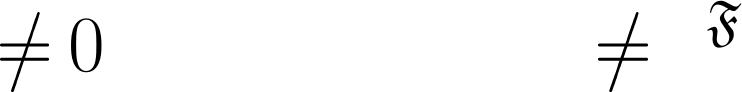Generalized proximities
The most natu ral way t o intr od uce func o ids is gener a lizing
proximity spaces.
Let δ be a proximity on a se t . It can be extended from
subsets of to ﬁlt ers on by the for mula
X δ
Y X X , Y Y: X δ Y .
I’ve pr oved that t here exist two functi o ns α : F() F()
and β: F() F() such that
X δ
Y Y α X
0
F()
X β Y
0
F()
.
10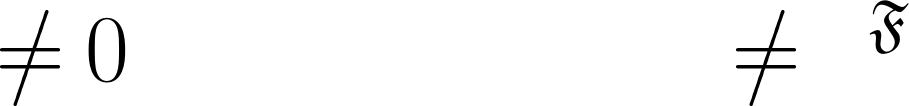Denit ion of funcoids
Let’s ﬁx two sets A and B.
The pair of two functions α: F(A) F(B) and β: F(B)
F(A) such that
Y α X
0
F(B)
X β Y
0
F(A)
denotes a funcoid. Strictl y speaking, a funcoid is a quad ruple
(A; B; α ; β) conforming to the above formula.
Thus func oids are a gener alization of proxim ity spac es.
I call funcoids (A; B; α; β) funcoids fro m A to B and denote
the s et of funco id s from A to B as FCD(A; B).
11
Source and destination of a f uncoid
The source an d the destination of a funcoi d f =(A; B; F ) are
Src f = A; Dst f = B.
12
Components of a funcoid: part 1
Let f = (A; B; α; β) be a fu ncoid. The n by
deﬁnition:
hf i = α.
A funcoid can be inverted (the invers e is al s o a fun coid):
f
1
= (B; A; β; α).
13
Components of a funcoid: part 2
We h ave
hf
1
i = β.
Thus a funcoid f ha s two component s :
hf i = α and hf
1
i = β.
An important property of funcoids : a funcoid f is completely
characterized by jus t one of its compone nts , s ay hf i. M ore-
over f is determined by values of hf i on p rincipal ﬁlters.
14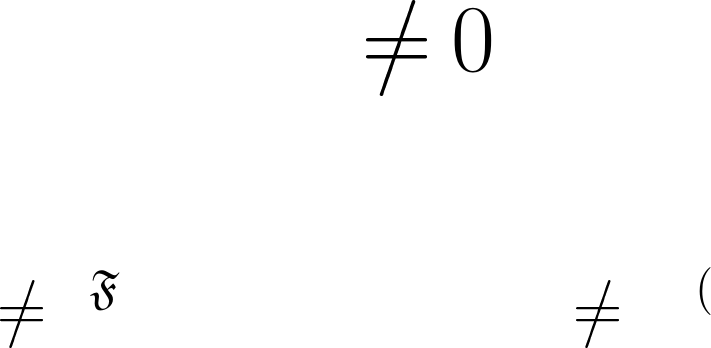Funcoids and r elations between lters
By deﬁnition
X [f ] Y Y hf iX
0
F(B)
.
We h ave
X [f ] Y Y hf iX
0
F(B)
X hf iY
0
F(A)
.
For brevity I will als o deﬁne:
hf i
X = hf i↑
A
X a nd X [f ]
Y
A
X [f ]
B
Y .
A funcoid f is completely characterized by the relation [f]
and even by hf i
or [f ]
.
15
Principal funcoids
Let A and B be sets.
For every bin a ry relat ion F P(A × B) the re exi s ts a
funcoid
FC D(A;B)
F FCD (A; B) d eﬁ ned by th e formula (for
every X PA)
h↑
FCD(A;B)
F i
X =
B
F [X].
This funco id is unique because a funco id is determined by
the values of its ﬁrst component on princ ip al ﬁlters.
I call funcoids corresponding to a binary relat io n by the for-
mula above as principal funcoids.
16
Funcoids & pre topol ogie s
Let α be a p r etopology, so α is a function F(). Th en
there exists a funcoid f such that
hf i
X =
G
{α(x) | x X }
(the join is taken on the lattice of ﬁlters).
So funcoids are a gene ralization of pretopologies.
17
Funcoids & pre closures
Let F be a precl o s ure (for example, F may be a topological
space in Kuratowski sense). Then there exists a funcoid f
such that
hf i
X =
B
F (X).
Thus func oids are a gener alization of preclosures.
18
Composi tion of funcoids
The compositio n of binary rel ations induces for principal
funcoids compo s ition wh ich complies wi th the formu la s :
hg f i = hgi hf i a nd h(g f)
1
i = hf
1
i hg
1
i.
We can deﬁ ne composition for funcoids by the same for-
mulas. Strictly speaking the compositio n of funcoids is
deﬁned by the formula:
(B; C; α
2
; β
2
) (A; B; α
1
; β
1
) = (A; C; α
2
α
1
; β
1
β
2
).
Composition of funcoids is assoc iative:
h (g f) = (h g) f.
19
Al ternate represe ntations of funcoids
Above I deﬁned fun co ids as quadruples. But a funco id can
be represented in two other ways:
as a binary relat ion δ P(PA × PB) between s et s
as a function α: PA F(B) from set s to ﬁlters
Below I will show the exact conditions required for δ an d
α in order t o represent a funcoid. Funco ids from A t o B
bijective ly correspond to such δ and α.
20
Funcoids as binary relations
A binary relation δ P(PA × PB) corresponds to a fun-
coid if and only if it com plies to the formu las (for all su itable
sets I, J, K):
¬( δ I); I J δ K I δ K J δ K;
¬(I δ ); K δ I J K δ I K δ J.
The funcoid f and r elation δ are related by the fo rmulas:
X [f ] Y X X , Y Y: X δ Y ;
X δ Y X [f ]
Y .
21
Funcoids as functions
A function α PA F(B) correspon ds to a f un coid if a nd
only if it complies to the formulas (for all sets I , J PA):
α = 0
F(B)
; α(I J) = αI αJ.
The funcoid f and f unction α ar e re la ted by the formulas:
hf iX =
l
{αX | X X };
αX = hf i
X.
22
Order of funcoids
The set FCD(A; B) of funcoids from A to B is a poset with
order de ﬁned by t he formula:
f g [f ][g].
More over it is a complete, di stributive, co-Brouwerian, ato m -
istic lattice.
23
Val ues of a join or meet of funcoids
For every R PFCD(A; B) and X PA, Y PB
1. X [
F
R]
Y f R: X [f]
Y ;
2. h
F
Ri
X =
F
{hf i
X | f R}.
For every R PFCD(A; B) and x, y being ultraﬁlters on A
and B correspond in g ly we have:
1. x [
d
R] y f R: x [f ] y;
2. h
d
Rix =
T
{hf ix | f R}.
24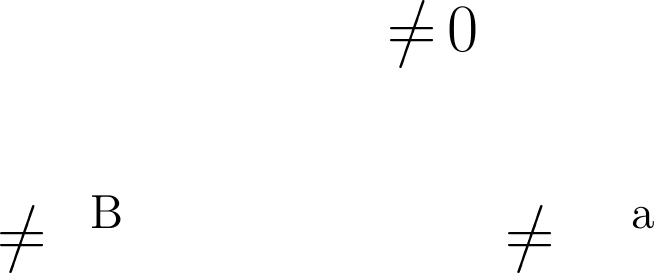Funcoidal product
The funcoidal product of ﬁlters is a generalization of the
Cartesian product of sets.
Let A and B be ﬁlters. Then there ex ists a uniq ue funcoid
(the funco i dal product of A and B) A ×
FCD
B such that
hA ×
FCD
BiX =
(
B if X A
0
Base(A)
;
0
Base(B)
if X A = 0
Base(A)
;
X [A ×
FCD
B] Y X A
0
Base(A )
Y B
0
Base(B)
.
25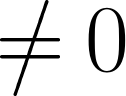Restricted identity funcoid
Restricted identity fun coids are a generalization o f identity
relations on a set.
Let A be a ﬁlter . T hen there exists a unique f uncoid (the
restri cted identity funcoid on A) id
A
FC D
such that:
hid
A
FCD
iX = X A;
X [id
A
FCD
] Y X Y A
0
Base(A)
.
26
Restriction of funcoids
Restriction of a funcoid f to a ﬁlte r A is deﬁned by the
formula
f |
A
=f id
A
FCD
.
It follows
f |
A
=f (A ×
FCD
1
F(Dst f )
).
27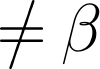T
0
-, T
1
- and T
2
-separable funcoids
A funcoid f is T
1
-separable when
α Src f , β Dst f : (α
β ¬ ({α} [f ]
{β })).
An endofun coid (a funco id with the same source and desti-
nation) i s :
1. T
0
-sepa rable when f f
1
is T
1
-sepa rable.
2. T
2
-sepa rable when f
1
f is T
1
-sepa rable.
28
Some properties of funcoids
Let f, g be f uncoids, X , Z be lt ers. Then X [g f] Z iﬀ
there exists an ultraﬁlter y su ch th at X [f] y and y [g] Z.
Let f, g, h be funcoids. Then
f (g h) = f g f h.
Also
f (A ×
FCD
B) = id
B
FCD
f id
A
FCD
.
29
Reloids
Reloids are a trivi a l generalization of uniform spaces.
Roughly spea king, a relo id is a ﬁlter on a Cart es ian produ ct
of two se ts.
To be precise, I deﬁne a reloid as a triple f = (A; B; F ) where
A and B are sets and F is a ﬁlter on A × B.
Note that reloids are also a generaliza ti on of binary rel a tions.
The reverse reloid f
1
is deﬁned as follows:
f
1
= (A; B; F )
1
= (B; A; F
1
).
I will also deno te GR(A; B; F ) = F .
30
Principal reloi ds
Let F be a bin a ry relat io n be tween sets A and B.
Then
RLD(A;B)
F = (A; B;
A×B
F )
is so called the principal reloid c orrespo nding to t he relation
F .
31
Composi tion of rel oids
Let f = (A; B; F ) a nd g = (B; C; G) be reloids. The
composition g f is deﬁned by the for mula
g f =
l
RLD(A;C)
(Y X) | X GR F , Y GR G
.
In other words, t he composition corresponds to t he ﬁlter (on
A × C) deﬁn ed by the b ase
{Y X | X GR F , Y GR G}.
Composition of reloids is associative.
32
Reloidal product
The reloidal product of ﬁlter s is a generalization of the Carte-
sian prod uct of s ets.
Let A and B be ﬁlters. Th en the reloid al prod uct of A a nd
B is deﬁned by the formu la:
A ×
RLD
B =
l
RLD(A;B)
(A × B) | A A, B B
.
In other words, the reloidal product is th e r eloid deﬁned by
the base
{A × B | A A, B B}.
33
Restricted identity reloid
The identity reloid on a set A is deﬁned as id
RLD(A)
=
RLD(A;A)
id
A
.
Similarly to the above deﬁned restricted ide ntity funcoid, we
can also deﬁne the restricted ident i ty reloid
i d
A
RLD
= id
RLD(Base(A ))
A ×
RLD
1
F(Base(A))
.
We h ave
id
A
RLD
=
l
RLD(Base(A);Base(A))
id
A
| A up A
.
34
Restriction of a reloid
Restriction of a reloid f to a ﬁlter A is deﬁned by the fo rmula
f |
A
=f id
A
or
f |
A
=f (A ×
RLD
1
F(Base(A))
).
35
Some properties of reloids
Let f, g, h be reloids. Then
f (g h) = f g f h.
Also
f (A ×
RLD
B) = id
B
RLD
f id
A
RLD
.
36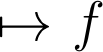Ordered and dagger categories
I call a partially ordered category a category together with a
partial order on each of its Hom-sets.
A d agger category is a category together with a function
f
f
on the set of morphisms which inver s es the source
and th e destination of the morphism and is subject to the
following cond it io ns:
1. f
††
= f ;
2. (g f)
= f
g
;
3. (1
A
)
= 1
A
.
37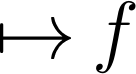Categor ies of funcoids and reloids
Funcoids with objects being sets and compositio n of f uncoids
form a category which I c a ll t he categ ory of funcoids.
The same holds for reloids.
Categories of funcoids and reloids are bo th pa rtially ordered
dagger catego ries with “th e dagger” deﬁned as
f
f
1
.
The orde r and the dag ger agree:
f
g
f g.
38
Special morphis ms
Let f be a morphism of a part ially ordered dagger category.
f is monovalued when f f
1
Dst f
.
f is entirely deﬁned when f
f 1
Src f
.
f is injective when f
f 1
Src f
.
f is s urjective when f f
1
Dst f
.
It’s easy to show t hat this is a generalization of monovalued,
entirely deﬁned, injective, a nd surjective b inary relations as
morph isms of the cat egory Rel.
39
Monovalued funcoids
I will denote “atoms a the set o f at oms under a for an ele-
ment a of a po s et.
The follow ing statements a re equiva lent for a funcoid f:
1. f is mo novalu ed.
2. a atoms 1
F(Src f )
: hf ia atoms 1
F(Dst f )
0
F(Dst f )
.
3. ∀I , J F(Dst f): hf
1
i(I J ) = hf
1
iI hf
1
iJ .
4. I , J P(Dst f): hf
1
i
(I J) = hf
1
i
I hf
1
i
J.
Consequently a principal funcoid is mon ovalued i its corre-
spondi ng binary relation is monovalued (a fun ct ion).
40
Functions between spaces
Let µ and ν be funcoids corres pond in g to a (pre )topological
spaces , or proximity spaces, or µ and ν be unifo rm spaces
(that is reloids ).
Let f be the funcoid or reloid correspo nding to a function
from the ﬁrst space to the sec o nd spac e.
41
Conti nuous morphis ms
Continui ty, p roximal cont inuity, an d uniform continuity of
f is e xp ressed by the sa m e formu la:
f µ ν f .
In the case if f is mon ovalued and entirely deﬁned, we have
f µ ν f µ f
1
ν f f µ f
1
ν.
This can be generalized for any partially ordered da gger cat-
egories.
42
Relationships of funcoids and rel oids
For every sets A, B we have FCD(A; B) and RLD(A; B)
interrela ted by the below deﬁn ed func ti o ns:
(FCD): RLD(A; B) FCD(A; B);
(RLD)
in
: FCD(A; B) RLD(A; B);
(RLD)
o ut
: FCD(A; B) RLD(A; B).
43
The funcoid induced by a reloid
Every reloid f RLD (A; B) induces a funcoid (FCD)f
FCD(A; B) by the fo llowing fo rmulas:
X [(FCD)f] Y F GR f : X
FCD(A;B)
F
Y;
h(FCD)f iX =
\

FC D (A;B)
F
X | F GR f
.
We h ave for every compo s able relo ids f and g:
(FCD)(g f) = ((FCD)g) ((FCD)f ).
I will skip some minor fact s on this topic.
44
The reloids induced by a funcoid
Every funco id f FCD(A; B) induces a reloid from A to B
in two ways, namely intersection o f outward relations and
union of in ward direct products of ﬁlter s:
(R LD)
out
f =
def
l
RLD(A;B)
[GR f];
(RL D)
in
f =
def
G
{A ×
RLD
B | A F(A), B F(B), A ×
FC D
B f }.
It’s simpl e to show that
(RL D)
in
f =
G
{a ×
RLD
b | a is an ato m of F(A ), b is an atom
of F(A), a ×
FCD
b f }.
I will skip some minor resu lts.
45
Some Galoi s connections
For every funcoid f we have
(FCD)(RLD)
in
f = f .
(FCD ): RLD(A; B) FCD (A; B) is the lower ad joint of
(RL D)
in
: FCD(A; B) RLD(A; B). Thus
1. (FCD)
F
S =
F
{(FCD)f | f S };
2. (RLD)
in
d
T =
d
{(RLD)
in
f | f T }
for every set S of reloids o r T of funcoids.
46
Convergence of funcoids
A ﬁlter F conve rges to a ﬁlt er A regarding to a funcoid
µ (F
µ
A) iﬀ F hµiA. (This generalizes the sta ndard
deﬁnition of ﬁlter convergent to a point or to a set.)
A funcoid f converges to a ﬁlter A regarding to a funcoid µ
(f
µ
A) iﬀ im f hµiA that is iﬀ im f
µ
A.
A funcoid f converges to a ﬁlter A on a ﬁlter B regarding to
a funcoid µ iﬀ f |
B
µ
A.
We can deﬁn e also convergence fo r a reloid f : f
µ
Aim f
hµiA or what is th e same f
µ
A (FC D)f
µ
A.
47
Limi t of a funcoid
lim
µ
f = a iﬀ
f
µ
Dst f
{a}
for a T
2
-sepa rable fu ncoid µ and a n on-empty funcoid f.
It is deﬁned correctly, that i s f h a s no more than one limit.
48
Generalized limit
We can deﬁne a (g en eralized) limit for an arbitrary (dis con-
tinuous ) functi o n, for exam ple any function on the set of
reals, or more generally fro m any topological vector space to
any t o pological vector space, etc.
An idea is th a t t he lim it should not change wh en translating
to a n other point of the spa ce. Thus we need to ﬁx a group
G of translations (or a ny other t ransformations) o f our sp ace.
49
Conditions for a general ized limit
Let µ an d ν be funcoids on a set , a nd G be a group o f
functions.
Let D be a s et such that
r G: im r D x, y Dr G: r(x) = y.
We require that µ and ever y r G co mmute, that is µ r =
r µ (for r considered as a princ ip al funcoid).
We r equire fo r every y
ν h ν i
{y} ×
FCD
hν i
{y}.
50
Denit ion of generalized l imit
xlim f =
def
{ν f r | r G}
for any funcoid f. (H er e G is consi dered as a se t of principal
funcoids.)
The limit at a point x is deﬁned as
xlim
x
f = xlim f |
hµi
{x}
.
51
Limi ts and gener alized limits
τ (y)=
def
{hµi{x} ×
FCD
hν i {y } | x D} .
Theorem Let µ be a T
2
-sepa rable funcoid and ν be a non-
empty funcoid such that ν ν ν. If lim
x
f = y then
xlim
x
f = τ (y).
This theore m establishes a bijective corres pondence (namely
τ) between limits and a subset o f gen eralized limits.
52
The skipped topics
In my speech I have skipped th e following topics:
specifyin g a fun coid by its values on ultraﬁlters
atomi c funcoids
complete funcoid s and reloids, complet ion of funcoids
and reloid s
conne ct edness of sets and of ﬁlters regarding funco ids
and reloid s
other
53
Further res earch direc tions
I also have researched pointfree funcoids, a generalizatio n of
funcoids relevant for po intfree topolog y, and mu ltifuncoids,
its f urther generalization.
The futu re research top ics includ e:
catego ries relat ed to funcoids
compactness o f funcoids
other
I have also formu la t ed a quite l arge number of o pen problems
related to ﬁlters, fu ncoids, and reloids. If you n eed a research
ﬁeld, I suggest you to solve my open problems.
I also have written a book.
54